# Clifford analysis

(diff) ← Older revision | Latest revision (diff) | Newer revision → (diff)

Clifford analysis studies functions with values in a Clifford algebra, and, as such, is a direct generalization to higher dimensions of the classical theory of functions of one complex variable (cf. Functions of a complex variable, theory of). It has its roots in quaternionic analysis, which was developed from the 1920s onwards as an, albeit modest, counterpart of the theory of two complex variables. The latter was to evolve into the vast and rich theory of several complex variables. The former gained renewed interest in the 1950s and led to hypercomplex function theory (cf. also Hypercomplex functions), renamed Clifford analysis in the 1980s, when it grew into an autonomous discipline.

Clifford algebra, also called geometric algebra, was introduced in 1878 by the English mathematician and philosopher W.K. Clifford, generalizing W.R. Hamilton's quaternions (cf. also Quaternion).

Ifis a real-dimensional (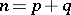) quadratic space with non-degenerate bilinear formof signature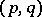, then, roughly speaking, the Clifford algebra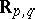over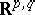is the real, associative and non-commutative algebra with identity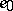, containingandas subspaces, and with a multiplication governed by the ruleIf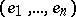is an orthonormal basis of, i.e.,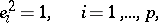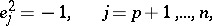then for vectors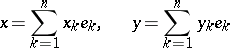in, their (geometric) product splits into a scalar and a so-called bivector part:where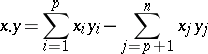and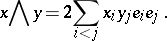Introducing conjugation as the anti-involution with, the squared length of a vector can be factorized asThis factorization implies the linearization of some fundamental partial differential operators of mathematical physics, such as the Laplace operator and the d'Alembert operator, and turns out to be crucial to Clifford analysis.

A general reference for Clifford algebra is [a4].

In the so-called Euclidean case, when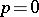and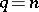, two fundamental differential operators are introduced: the Euclidean Dirac operator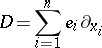and the Euclidean Cauchy–Riemann operator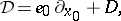the latter being a generalization of the classical Cauchy–Riemann operator which is obtained for, identifyingwith the imaginary unit (cf. also Cauchy–Riemann equations). These operators linearize the Laplace operator in the sense that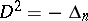and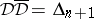.

Clifford analysis essentially deals with the study of null solutions of the operatorsand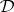, both cases showing many similarities, at least formally, as shown in [a2].

A function, continuously differentiable in an open domainofand taking values in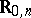, is said to be monogenic inif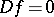in.

Monogenicity thus leads to an overdetermined first-order system, generalizing the Cauchy–Riemann system. In particular, ifis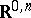-valued, the so-called Riesz system is obtained.

If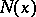is a fundamental solution of the Laplace operator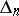, thenis an-valued fundamental solution of.

The power of classical function theory of one complex variable is based on the Cauchy integral formula (cf. Cauchy integral theorem) and the Weierstrass convergence theorem (cf. Weierstrass theorem) on the one hand, and on Riemann's concept of conformal mapping on the other; they constitute the nuclei of the so-called analytic and geometric function theories, respectively.

In their turn, the basic analytic properties of monogenic functions are based upon the Cauchy formula,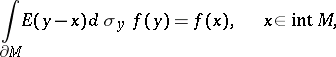whereis monogenic in,is a compact-dimensional manifold with boundary in, andis the vector-valued-dimensional surface element given by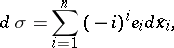References for function-theoretic results in Clifford analysis are [a1] and [a2].

Among others, the Cauchy operator associated with the Dirac operator, as defined by the left-hand side of Cauchy's formula, is used in studying boundary value problems in domains of.

The Cauchy operator associated with the Cauchy–Riemann operator, when applied to functions in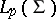(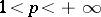), gives rise to the Cauchy transforms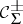and the singular Cauchy transform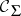on the Lipschitz surface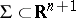, i.e. on a surface of the form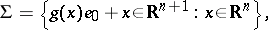where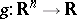is a Lipschitz function, possibly satisfying additional technical conditions (cf. also Lipschitz condition). It turns out that the scalar part ofis the classical singular double-layer potential operator on. As, moreover, the projection operatorsdetermine the Hardy spacesand the Hardy decomposition of, the singular Cauchy transformmay be viewed as a generalization of the classical Hilbert transform on the real line. These results reveal the potentials of Clifford analysis for dealing with problems of harmonic analysis in Euclidean space.

References for this area are [a3] and [a5].

Regarding the geometric viewpoint in Clifford analysis, Möbius transforms incan be completely characterized by specific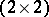-matrices over the Clifford group, the so-called Vahlen matrices. By means of this characterization, transforms of metrics and operators may be calculated. The introduction of Vahlen matrices has even inspired the recent development of Clifford analysis on hyperbolic spaces.

A reference for this area is [a4].

How to Cite This Entry:
Clifford analysis. Encyclopedia of Mathematics. URL: http://encyclopediaofmath.org/index.php?title=Clifford_analysis&oldid=15913
This article was adapted from an original article by F. Brackx (originator), which appeared in Encyclopedia of Mathematics - ISBN 1402006098. See original article